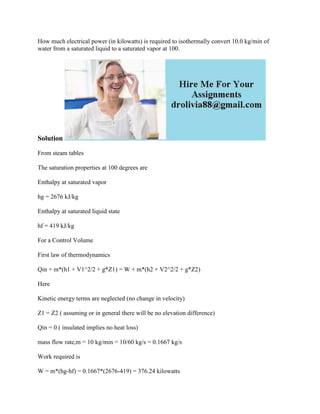Diese Präsentation wurde erfolgreich gemeldet.

# How much electrical power (in kilowatts) is required to isothermally c.docx

Anzeige
Anzeige
Anzeige
Anzeige
Anzeige
Anzeige
Anzeige
Anzeige
Anzeige
Anzeige
Anzeige×

1 von 2 Anzeige

# How much electrical power (in kilowatts) is required to isothermally c.docx

How much electrical power (in kilowatts) is required to isothermally convert 10.0 kg/min of water from a saturated liquid to a saturated vapor at 100.
Solution
From steam tables
The saturation properties at 100 degrees are
Enthalpy at saturated vapor
hg = 2676 kJ/kg
Enthalpy at saturated liquid state
hf = 419 kJ/kg
For a Control Volume
First law of thermodynamics
Qin + m*(h1 + V1^2/2 + g*Z1) = W + m*(h2 + V2^2/2 + g*Z2)
Here
Kinetic energy terms are neglected (no change in velocity)
Z1 = Z2 ( assuming or in general there will be no elevation difference)
Qin = 0 ( insulated implies no heat loss)
mass flow rate,m = 10 kg/min = 10/60 kg/s = 0.1667 kg/s
Work required is
W = m*(hg-hf) = 0.1667*(2676-419) = 376.24 kilowatts
.

How much electrical power (in kilowatts) is required to isothermally convert 10.0 kg/min of water from a saturated liquid to a saturated vapor at 100.
Solution
From steam tables
The saturation properties at 100 degrees are
Enthalpy at saturated vapor
hg = 2676 kJ/kg
Enthalpy at saturated liquid state
hf = 419 kJ/kg
For a Control Volume
First law of thermodynamics
Qin + m*(h1 + V1^2/2 + g*Z1) = W + m*(h2 + V2^2/2 + g*Z2)
Here
Kinetic energy terms are neglected (no change in velocity)
Z1 = Z2 ( assuming or in general there will be no elevation difference)
Qin = 0 ( insulated implies no heat loss)
mass flow rate,m = 10 kg/min = 10/60 kg/s = 0.1667 kg/s
Work required is
W = m*(hg-hf) = 0.1667*(2676-419) = 376.24 kilowatts
.

Anzeige
Anzeige

### How much electrical power (in kilowatts) is required to isothermally c.docx

1. 1. How much electrical power (in kilowatts) is required to isothermally convert 10.0 kg/min of water from a saturated liquid to a saturated vapor at 100. Solution From steam tables The saturation properties at 100 degrees are Enthalpy at saturated vapor hg = 2676 kJ/kg Enthalpy at saturated liquid state hf = 419 kJ/kg For a Control Volume First law of thermodynamics Qin + m*(h1 + V1^2/2 + g*Z1) = W + m*(h2 + V2^2/2 + g*Z2) Here Kinetic energy terms are neglected (no change in velocity) Z1 = Z2 ( assuming or in general there will be no elevation difference) Qin = 0 ( insulated implies no heat loss) mass flow rate,m = 10 kg/min = 10/60 kg/s = 0.1667 kg/s Work required is W = m*(hg-hf) = 0.1667*(2676-419) = 376.24 kilowatts